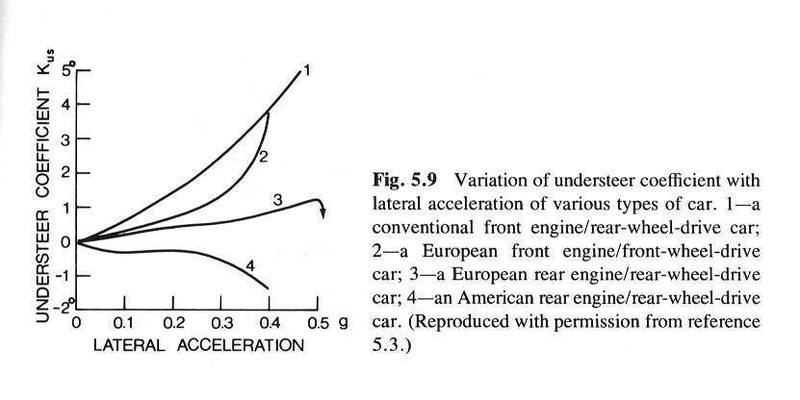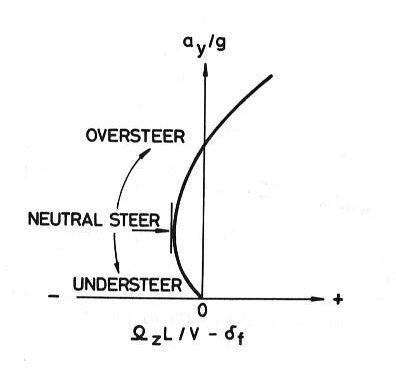# Steering angle for Autonomous vehicle

• alex1994

#### alex1994

Dear All,
Hello,
I'm studying electronics engineering and I'm new in vehicle dynamics,
Recently, I make an autonomous vehicle for my final project. In order to control steering angle of vehicle, I have difficulty to measure the steering angle based on curvature road and vehicle velocity.
Anybody can help me, how to calculate steering angle when wheelbase, curvature road and vehicle are already known?

Thanks

Thanks @billy_joule
am I not wrong steering angle (δ) can be determined by δ=L/R
where
L = Wheelbase
But there isn't relation with the speed.
is the steering angle is same both at high or low speed?

is the steering angle is same both at high or low speed?
Generally, yes.

Steering angle usually varies with lateral acceleration ($a_y$), which is related to speed ($v$) and radius of curvature ($R$) with the following equation: $a_y = \frac{v^2}{R}$.

The steering angle ($\delta$), lateral acceleration (related to standard gravity $g$) and wheelbase ($L$) are related the following way:
$$\delta = \frac{L}{R} + K_{us}\frac{a_y}{g}$$
Where $K_{us}$ is the understeer coefficient of the vehicle. If $K_{us} = 0$ then the vehicle is said to be «neutral steer» (steering angle independent of lateral acceleration); if $K_{us} < 0$ then the vehicle is said to be «oversteer».

Theoretically, based on the bicycle model, $K_{us} = \frac{W_f}{C_f} - \frac{W_r}{C_r}$, where $W$ is the normal weight acting on a tire and $C$ is the cornering stiffness of the tire ($f$ & $r$ subscripts are for «front» and «rear»). The cornering stiffness relates lateral tire force to slip angle.

In practice, $K_{us}$ for a given vehicle varies with lateral acceleration. The way to determine $K_{us}$ for a given vehicle following a path is by plotting the variable $\frac{a_y}{g}$ with respect to $\frac{L}{R} - \delta$ and evaluate:
$$\frac{d\left(\frac{a_y}{g}\right)}{d\left(\frac{L}{R} - \delta\right)} = - \frac{1}{K_{us}}$$
From measurements taken within the vehicle, $R = \frac{v}{\Omega_z}$. Where $\Omega_z$is the yaw velocity of the vehicle.

Ref.: http://ca.wiley.com/WileyCDA/WileyTitle/productCd-0470170387.html, 2nd ed. by J.Y. Wong•billy_joule